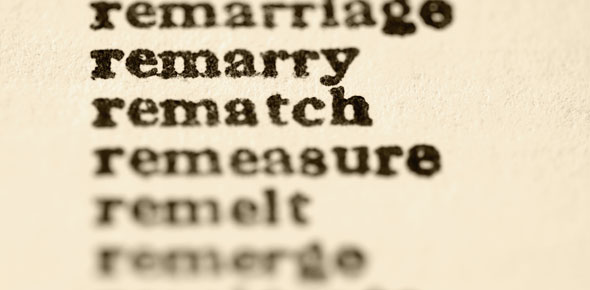# Vocabulary 5: Circles

32 Questions | Total Attempts: 13SettingsThis quiz contains 35 defintions relating to circles that you might find on future tests, though few are likely to be on the final.  Here you need to provide the correct geometric term to match the definition.  Spelling counts.  Good luck !

Related Topics
• 1.
The linear distance representing the arc.  That part of the circumference proportional to the measure of the central angle when compared to the entire circle.
• 2.
Either of the two parts into which a circle is divided by a line containing the diameter of the circle.
• 3.
Two arcs of a circle that have exactly one point in common.
• 4.
An unbroken part of a circle.
• 5.
For a minor arc, the degree measure of its central angle.  For a major arc, 360 minus the degree measure of its central angle.  For a semicircle, 180.
• 6.
A minor arc that has the same endpoints as the chord.
• 7.
A point from which all given points in the circle are equidistant.
• 8.
For a given circle, an angle that intersects the circle in two points and has its vertex at the center of the circle.
• 9.
For a given circle, a segment whose endpoints are points on the circle.
• 10.
A set of points that consists of all points in a plane that are a given distance from a given point in the plane, called the center.
• 11.
With respect to a polygon and a circle, a polygon such that each side of the polygon is tangent to the circle.
• 12.
Circles that lie in the same plane and have the same center but have different radii.  Yes they're similar, but what else are they?
• 13.
Two arcs of the same or congruent circles that have the same measure.
• 14.
Circles that have the same radius.
• 15.
In a circle, a chord that contains the center of the circle.
• 16.
An angle whose vertex lies on a given circle and whose sides are two chords of the circle.
• 17.
With respect to a polygon and a circle, a polygon such that each of its vertices lies on the circle.
• 18.
With respect to an angle and an arc, an arc such that: (1) The endpoints of the arc lie on the angle, (2) All points of the arc except the endpoints are in the interior of the angle, and (3) each side of the angle contains an endpoint of the arc.
• 19.
For and angle and an arc, an arc such that if ےAPB is a central angle of circle P, and C is any point on the circle and in the exterior of the angle, then points A and B and all points of the circle exterior to ےAPB form an arc that includes C, called ACB.
• 20.
For an angle and an arc, an arc such that if ےAPB is a central angle of circle P, and C is any point on the circle and in the exterior of the angle, then points A and B and all points of the circle interior to ےAPB form an arc, called AB, which does not include C.
• 21.
The ratio of the circumference of a circle to its diameter.
• 22.
For a line that intersects a circle at only one point, the point at which they intersect.
• 23.
A segment whose endpoints are the center of the circle and any point on the perimeter.
• 24.
For a circle, a line that intersects the circle in exactly two points or a segment that intersects the cricle in two points and extends outside the circle.
• 25.
Circles that have different radii and may have different centers.
• 26.
A line in the plane of the circle that intersects the circle in exactly one point.
• 27.
A region bouded by the central angle and its intercepted major or minor arc.
• 28.
All points such that the circumference is between the point and the center of the circle.
• 29.
The part of a secant segment that is exterior to the circle.
• 30.
A segment from a point exterior to the circle to a point on the circle and containing a chord of the circle.
• 31.
A segment AB such that one endpoint is on a circle, the other is outside the circle, and the line AB is tangent to the circle.
• 32.
An equation of the form (xa) 2 + (yb) 2 = k2, which shows all the points a distance of k units from a center at (a, b).  Note that a circle is not a linear function, because it has more than one y value for most values of x